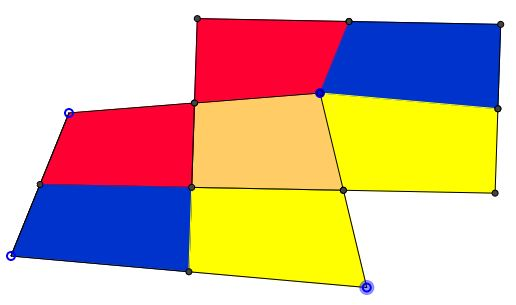### Problem

For every (convex, and, if so, for non-convex) quadrilateral ($ABCD$ below) there is a parallelogram ($OO'B'O''$) below of the same area but smaller perimeter.### Solution

The proof depends on the following property of bimedians - the lines joining the midpoints of the opposite sides of a quadrilateral.

In a quadrilateral $ABCD,$ $E$ is the midpoint of $AD,$ $G$ the midpoint of $BC.$Then $2EG\le GK+KS=GK+GL= AB+CD.$

Apply that to the diagram below:$2OO'=2F'H\le AD+BC.$

And, similarly,

$2OO''=2EG\le AB+CD.$

Note that, by the construction, $OO'B'O''$ is a parallelogram with perimeter

$2(OO'+OO'')\le AD+BC+AB+CD,$

the perimeter of $ABCD.$ The construction (by dissection and rearrangement) makes it obvious that the two quadrilaterals have the same area.

• Isoperimetric Theorem and Inequality
• An Isoperimetric theorem
• Isoperimetric theorem and its variants
• Isoperimetric Property of Equilateral Triangles
• Isoperimetric Property of Equilateral Triangles II
• Maximum Area Property of Equilateral Triangles
•